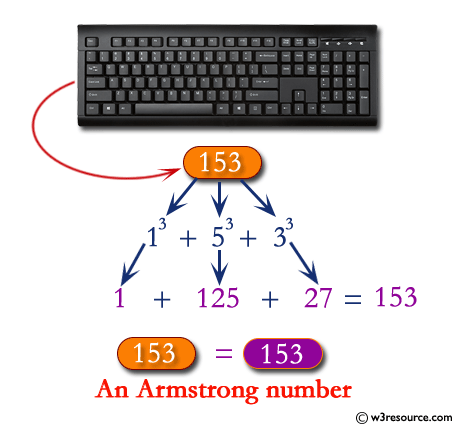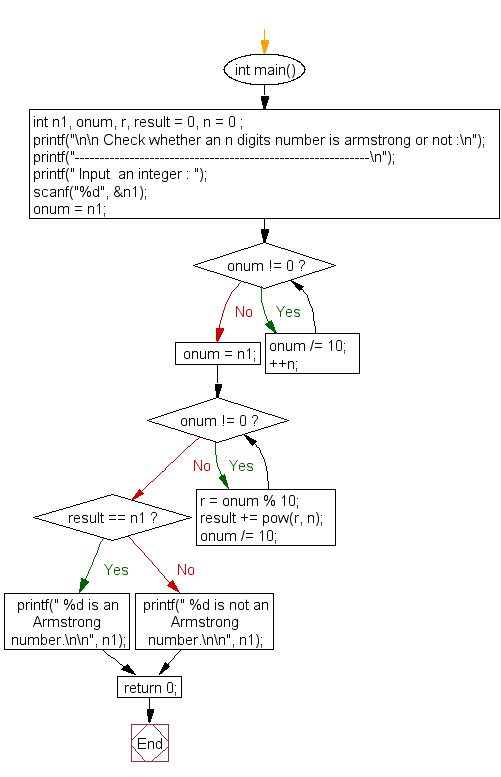﻿ C : Check whether an n digits number is armstrong or not# C Exercises: Check whether an n digits number is Armstrong or not

## C For Loop: Exercise-59 with Solution

Write a program in C to check Armstrong number of n digits.

Pictorial Presentation:Sample Solution:

C Code:

``````#include <stdio.h>
#include <math.h>

int main()
{
int n1, onum, r, result = 0, n = 0 ;
printf("\n\n Check whether an n digits number is armstrong or not :\n");
printf("-----------------------------------------------------------\n");

printf(" Input  an integer : ");
scanf("%d", &n1);

onum = n1;

while (onum != 0)
{
onum /= 10;
++n;
}

onum = n1;

while (onum != 0)
{
r = onum % 10;
result += pow(r, n);
onum /= 10;
}

if(result == n1)
printf(" %d is an Armstrong number.\n\n", n1);
else
printf(" %d is not an Armstrong number.\n\n", n1);

return 0;
}
```
```

Sample Output:

``` Check whether an n digits number is Armstrong or not :
-----------------------------------------------------------
Input  an integer : 1634
1634 is an Armstrong number.
```

Flowchart:C Programming Code Editor:

Improve this sample solution and post your code through Disqus.

What is the difficulty level of this exercise?

Test your Programming skills with w3resource's quiz.

﻿